# Introduction

Interpolating polynomials are not very good at approximating discontinuous functions—the error analysis specifically requires a certain amount of continuity. Unfortunately, most sampled data is associated with random noise which is, by its nature, non-differentiable. As a consequence, using polynomial interpolation will result in significant errors espcially as the number of points goes up.

# Background

Useful background for this topic includes:

• Vandermonde Matrix

# References

• Walter Rudin, Principles of Mathematical Analysis, 3rd Ed., McGraw Hill, 1976.

# Theory

In this topic, we will look at the effects on polynomial interpolation by various features which may cause significant sources of error. The resulting lack-of-accuracy is often termed polynomial wiggle.

## The Approximation Theorem of Weierstrauss

Werestrauss's approximation theorem states that for any continuous function defined on a closed interval [a, b], there exists a sequence of polynomials which converge uniformly to the function. While this does not guarantee that there exists interpolating polynomials (the proof uses Bernstein polynomials which are fixed at the end points), it at least provides a basis for approximating functions using interpolating polynomials. We will see, however, that there are problems.

## Discontinuities

Polynomials are very poor at interpolating discontinuities: any reasonable error analysis cannot be performed due the lack of continuity. To demonstrate this, Figures 1 and 2 show the interpolation of the unit step function with 5 and 7 points, respectively.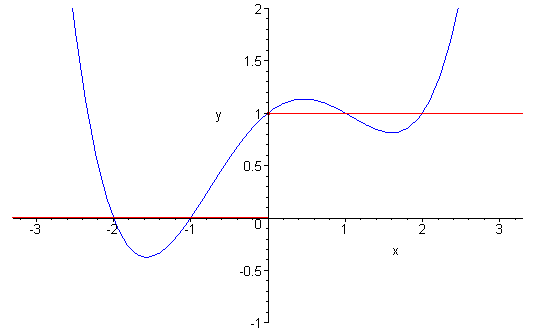Figure 1. Interpolating the unit step function with 5 points.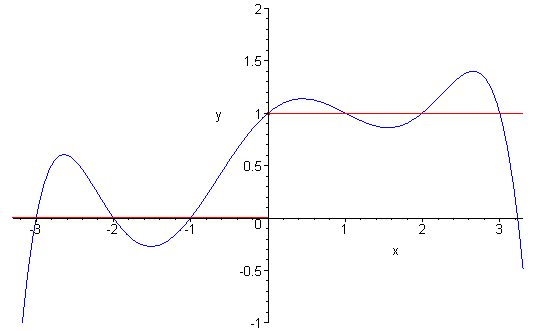Figure 2. Interpolating the unit step function with 7 points.

## Non-Linearity

A classic example of a function which cannot be easily interpolated is the Gaussian function e-x2. The coefficients of the Taylor series of this function do not drop off as quickly as they do with other more familiar functions such as the simple exponential or other trigonometric functions. Consequently, interpolating polynomials produce less than desirable results unless the points are very close to each other. Figure 3 shows the Gaussian function interpolated with 5 and 7 points.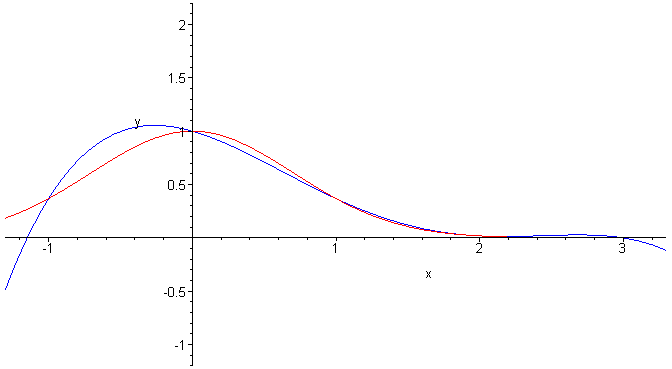Figure 3. Interpolating the Gaussian function with 5 points.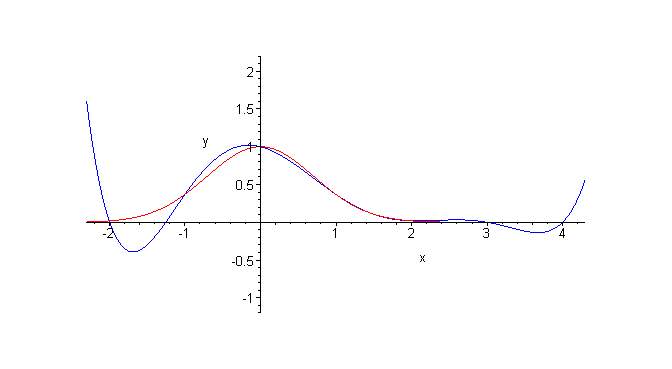Figure 4. Interpolating the Gaussian function with 7 points.

## Errors in Points

The exponential function is sufficiently well behaved that interpolating polynomials provide a reasonable fit, as is demonstrated in Figures 5 and 6 which plot the function and its approximation, and the error of the approximation for a polynomial of degree 7 interpolating the eight points 0, 1, 2, ..., 7.Figure 5. Interpolating the exponential function with 8 points.Figure 6. The difference between the interpolating polynomial and the exponential function on [0, 7].

The points being interpolated are

1, 0.3679, 0.1353, 0.04979, 0.01832, 0.006738, 0.002479, 0.0009119

and the demonstrate the fit, Figure 7 focuses on tail of the plot.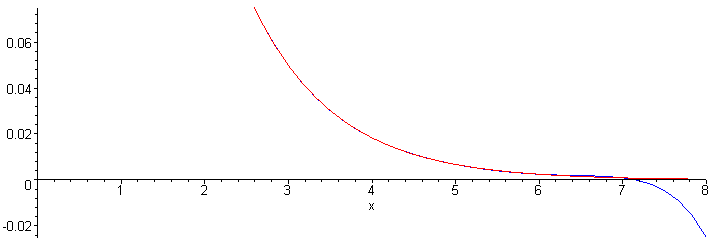Figure 7. A zoom of the ordinate axis to show the closeness.

The points

1.035, 0.3410, 0.1422, 0.04621, 0.01776, 0.006648, 0.002676, 0.0009092

are the eight above values together with an introduced relative error of no greater than 10% for each point (the errors were randomly generated by Maple). The small errors result in a significant change to the interpolating polynomial as is shown in Figure 8 which shows the polynomial interpolating the points which have noise associated with them.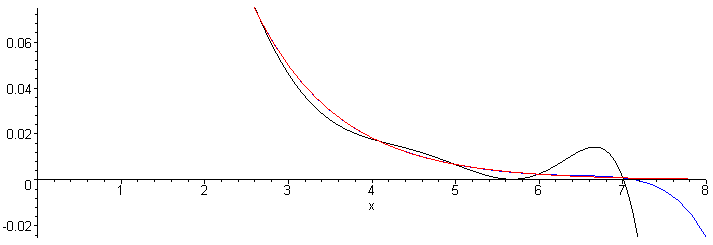Figure 8. A zoom of the exponential function, a polynomial interpolating the exact values, and the polynomial interpolating noisy values.

## Error and Spacing

The last example demonstrated how a small amount of noise can result in a significant reduction in the accuracy of the interpolating polynomial; however, with lower degree polynomials this is of less significance.

When interpolating two points, the error is propagated linear, as is shown in Figure 9 which shows a family of interpolating polynomials around the points (1, 5±0.1) and (2, 3±0.1).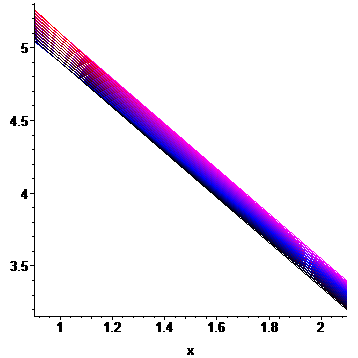Figure 9. Interpolating lines between points (1, 5±0.1) and (2, 3±0.1).

If three points are interpolated with a quadratic and the points are evenly spaced, errors in the y values do not propagate too significantly, as is shown in Figure 10. In this case, the interpolating polynomial is p(x) = 0.5 x2 − 3.5 x + 8.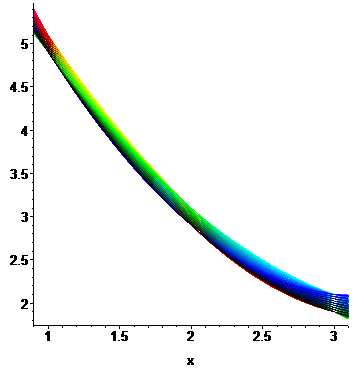Figure 10. Interpolating quadratic polynomials between points (1, 5±0.1), (2, 3±0.1), and (3, 2±0.1).

Figure 11 takes the same polynomial found to fit the points (1, 5), (2, 3), and (3, 2); evaluates it at 2.5; and then interpolates the three points (1, 5±0.1), (2.5, 2.375±0.1), and (3, 2±0.1). As can be seen, there is a much greater variation in the possible number of interpolating polynomials.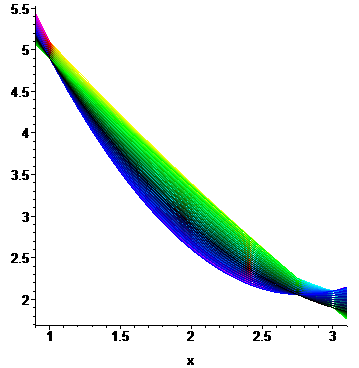Figure 11. Interpolating quadratic polynomials between points (1, 5±0.1), (2, 3±0.1), and (3, 2±0.1).

The effect becomes even more obvious when choosing the points (1, 5±0.1), (2.9, 2.055±0.1), (3, 2±0.1) as is shown in Figure 12.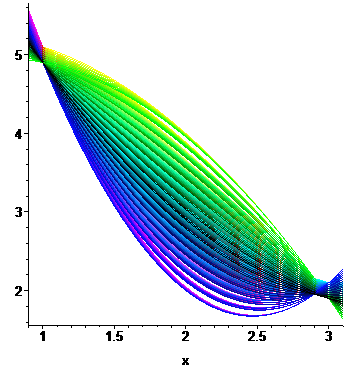Figure 12. Interpolating quadratic polynomials between points (1, 5±0.1), (2, 3±0.1), and (3, 2±0.1).

This can be seen by looking at the error for interpolating polynomials (as described in the topic on the Vandermonde Matrix); however, these images are presented here to emphasize the problems: For data with noise, the points should not be too close, otherwise the error will be significantly magnified even for lower-order interpolating polynomials.

## Runge's Phenomenon

The previous section demonstrates that spacing must be taken into consideration; however, Runge's phenomenon shows that equal spacing is not appropriate, either. The interpolating polynomials of the function f(x) = 1/(1 + 25x2) which sample the function at equally spaced points on the interval [-1, 1] do not converge to the function but instead oscillate more-and-more wildly towards the boundaries. The interpolating polynomials of the aforementioned function are shown in Figure 13. See the discussion on error analysis for one method to correct this.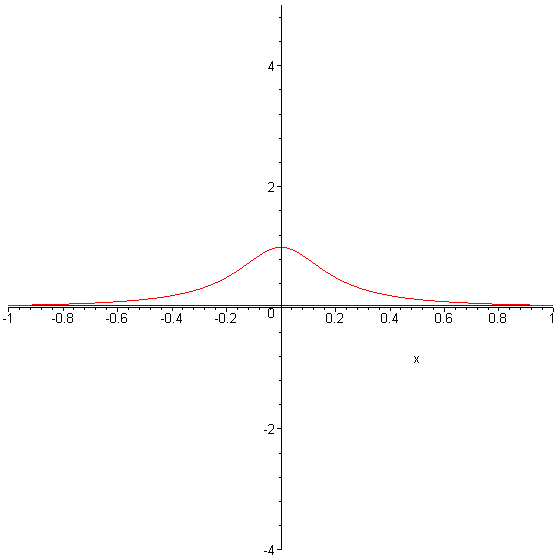Figure 13. Interpolations of equally spaced points to f(x) = 1/(1 + 25x2) (click for more iterations).

## Oscillating Functions

Interpolating oscillating functions can also lead to erroneous interpolating functions. Figures 14, 15, and 16 demonstrate how interpolating seven points -2, -1, 0, 1, ..., 4 on the functions sin(x), sin(2x), and sin(4x) can yeild either a very good approximation or a very poor approximation.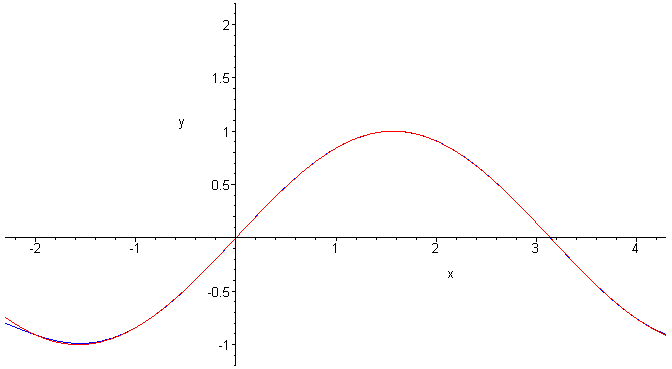Figure 14. Interpolating seven points on sin(x).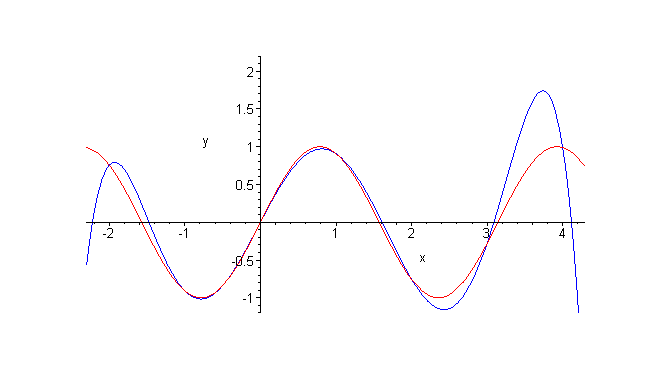Figure 15. Interpolating seven points on sin(2x).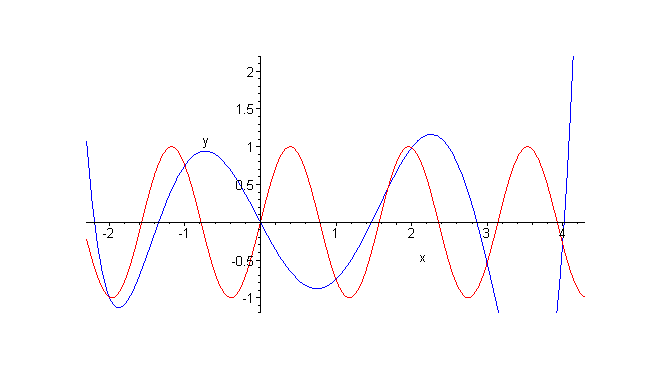Figure 16. Interpolating seven points on sin(4x).

If the data comes from a system which is known to be sinusoidal with a known frequency ω, it would be better to interpolate be better to use an interpolating function of the form f(x) = c1sin(ωx) + c2cos(ωx).

# HOWTO

Polynomial interpolation should not be used if the points come from a function which is discontinuous and oscillating functions should be sampled at a rate at least four times the frequency (at least for polynomial interpolating). Caution should be exercised when interpolating data with noise: the greater the noise, the lower the degree of any interpolating polynomial and care should be taken that the x-values are not too close but that equally spaced x-values may also cause problems for very high degree interpolating polynomials.

# Error Analysis

This topic has discussed a number of the problems which are associated with polynomial interpolation. These are given the rather humorous name polynomial wiggle which suggests the effects of the interpolating polynomials.

One observation which may be made by looking at a polynomial with equally spaced roots, as shown in Figure 17. The error of an interpolating polynomial on nine points depends on this function and it is clear that the error are more magnified at the ends and the error is suppressed near the middle.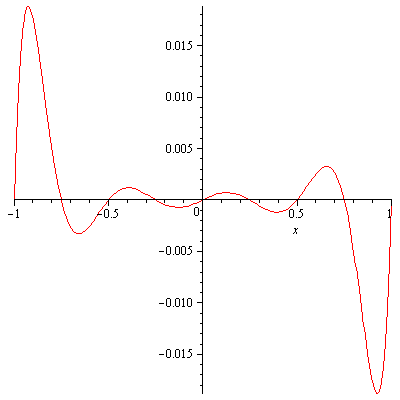Figure 17. The error of an interpolating polynomial with 9 equally spaced points.

One possible solution is to more densely pack the interpolating points closer to the boundaries. While the theory is beyond the scope of this text (for now), it can be shown that if the points are chosen according to the roots of a Chebyshev polynomial T9(x), the error is reduced uniformly, as is shown in Figure 18.Figure 18. The error of an interpolating polynomial with 9 points spaced according to the roots of the Chebyshev polynomial T9(x).

# Maple

The following Maple commands find the roots of the nth Cheybshev polynomial:

```> ChebyshevT( 5, x );
ChebyshevT(5, x)

> expand( % );
5       3
16 x  - 20 x  + 5 x

> fsolve( % );
-0.9510565163, -0.5877852523, 0., 0.5877852523, 0.9510565163

> ChebyshevT( 7, x );
ChebyshevT(7, x)

> expand( % );
7        5       3
64 x  - 112 x  + 56 x  - 7 x

> fsolve( % );
-0.9749279122, -0.7818314825, -0.4338837391, 0., 0.4338837391, 0.7818314825, 0.9749279122
```